International Journal of Intelligent Information Systems
Volume 5, Issue 1, February 2016, Pages: 1-4

Maximum Power Point Tracking (MPPT) Using Artificial Bee Colony Based Algorithm for Photovoltaic System

Hassan Salmi*, Abdelmajid Badri, Mourad Zegrari

EEA&TI Laboratory, Faculty of Sciences and Techniques, Hassan II Casablanca University, Mohammedia, Morocco

Email address: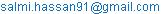(H. Salmi)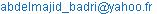(A. Badri)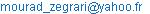(M. Zegrari)

To cite this article:

Hassan Salmi, Abdelmajid Badri, Mourad Zegrari. Maximum Power Point Tracking (MPPT) Using Artificial Bee Colony Based Algorithm for Photovoltaic System. International Journal of Intelligent Information Systems. Vol. 5, No. 1, 2016, pp. 1-4. doi: 10.11648/j.ijiis.20160501.11

Abstract: The Artificial Bee Colony based algorithm (ABC) studied in this paper is assigned as an intelligent control of photovoltaic system. The output power of a photovoltaic panel depends on solar irradiation and temperature. Therefore, it is important to operate the photovoltaic (PV) panel in its maximum power point. In this aim, the ABC consists to track the optimal duty cycle of the electronic converter, in order to lead to the Maximum Power Point (MPP) of the PV system. Moreover, the classical method Perturb and Observe (P&O) [1-2] is studied in the sake of comparison with the ABC method in Matlab/Simulink, by taking into consideration the efficiency, the speed and the robustness performance when the meteorological conditions change.

Keywords: Maximum Power Point, Artificial Bee Colony, P&O, Matlab/Simulink

Contents

1. Introduction

Photovoltaic (PV) systems have generated immense market and research interests recently due to the abundance of raw materials and their noiseless and environment friendly power-generating process [3-4]. The electrical energy produced by a photovoltaic system stored or used directly by static converters is not maximized. The maximum power point (MPP) is achieved by adjusting the operating point of the PV array using a DC–DC converter. In this context, an increasing interest has been given to the methods of tracking the maximum power of the PV system named Maximum Power Point Tracker (MPPT) .

Different techniques to track the MPP have been presented in literatures. These techniques are dedicated to find the optimal duty cycle of the boost, we can distinguish the conventional P&O which known by oscillation in the steady state and the inability to provide the appropriate MPP when the meteorological condition change. In this work an intelligent approach using Artificial Bee Colony has introduced to track the maximum power point of the photovoltaic panel.

We studied the methods of P&O and ABC in order to compare the performances. The results are obtained using Simulink environment. The system is presented in Fig. 1.

This paper is organized as follows: In section II, description of the photovoltaic system. Maximum power point tracking control is described in section III. The simulation and results are presented in section IV. Conclusion is given in the last section.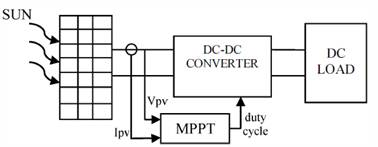Figure 1. General diagram of the photovoltaic system.

2. Description of the Photovoltaic System

2.1. Characteristics of PV Module

A PV array is formed of several PV modules connected in series and/or parallel and the total power is a combination of the power derived from each PV module. . The fundamental equation for PV panel is given by: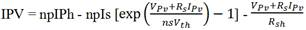(1)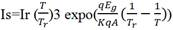(2)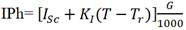(3)

where:

Table 1. Parameters of a PV.

 IPV Output current VPV Output voltage T Cell temperature (K). G Solar irradiance (W/m2). IPh Light-generated current. Is PV cell saturation current. Ir saturation current at Tr. Isc Short-circuit current at reference condition. Tr Reference temperature. KI Short-circuit temperature coefficient. q Charge of an electron. K Boltzmann's constant. Eg Band-gap energy of the material. A Ideality factor.

The behavior of the PV module is shown in two characteristics IPV(VPV) and PPV(VPV) for different irradiation and temperature values can be plotted.

The following curves give an example of these characteristics for two different irradiance (G) and temperature: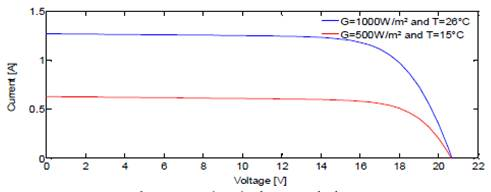Figure 2. PV Characteristics of the photovoltaic Panel IPV(VPV).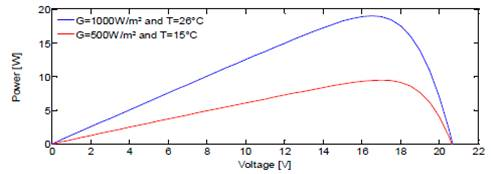Figure 3. P(VPV) Characteristic.

The specification of the photovoltaic module used are:

Table 2. The specification of the photovoltaic module used.

 Pmax (W) 20.0W. Voc (V) 20.8V. Isc (A) 1.27A. Vmp(V) 17.1V. Imp(A) 1.17A.

2.2. Boost DC-DC

A boost chopper is one of power converters used in photovoltaic systems. In this aim we will insert this converter 'boost' between the photovoltaic system and the load in order to bring this load to function optimally. The control of the converter is managed by the duty cycle which itself needed to be adjusted to its optimal value. This adjustment is actually directed by MPPT methods. The boost converter is composed of inductor, capacitor, and a power switch often is a mosfet transistor. The boost circuit is depicted in Fig. 4 .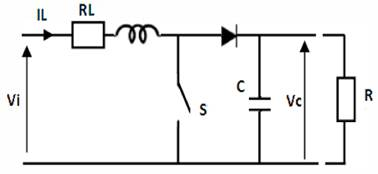Figure 4. Boost Converter circuit.

2.3. Maximum Power Point Tracking

Maximum power point tracking (MPPT) technique is used to extract the maximum power delivered by the solar panel. Indeed there are several algorithms to extract the maximum power. The most famous is Perturb & Observe (P & O).In this section we offer a new controller based on ABC.

1)  Perturb and Observe:

Perturb and observe approach is the most used method to pursue the maximum power point of a photovoltaic generator. The principle is to disrupt the voltage or duty cycle and calculating the power terminal of the PV module.

When p(k-l)<p(k), the voltage is increased.

When p(k-l) > p(k), the voltage is decreased.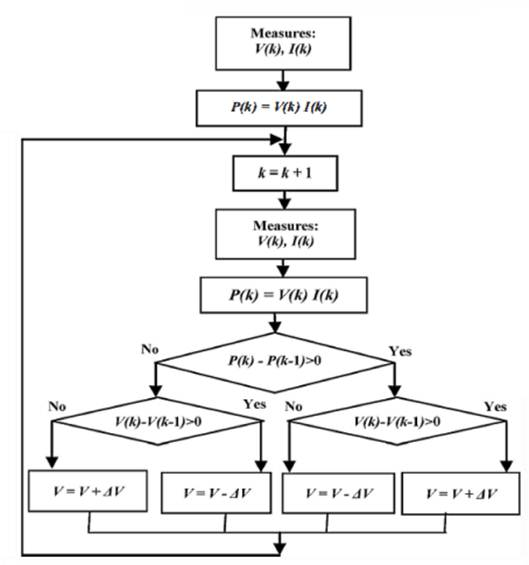Figure 5. Flowchart for conventional Perturb and Observe algorithm.

2)  Principle of ABC algorithm:

The artificial bee colony (ABC) algorithm was introduced for solving optimization problems proposed by Tereshko and Loengarov .

The ABC consists of three groups of artificial bees: employed, onlooker and scouts. Every group has a different task in the optimization process .

The employed bee is currently searching for food and carry the data about food source back to the hive. A bee waiting in the hive for making decision to select a food source is called as an onlooker. Whenever a food source is exploited fully, all the employed bees associated with it abandon the food source, and become scout. The number of food sources is equal to the number of employed bees and also equal to the number of onlooker bees. The flowchart of the proposed ABC algorithm is given in Fig. 6.

The steps of the ABC algorithm are outlined as follows:

1. At the initialization phase, the ABC generates a randomly distributed initial population of ns solutions (duty cycle). Each solution is produced within its limits [0, 1] according to the equation below:

Xj = Xmin + rand[0, 1]*( Xmax- Xmin) i=1, 2,…., SN    (4)

Where xmin and xmax represent respectively the minimum and the maximum of the parameter j.

2. Each employed bee leaves to a food source and locates a neighbor source vi according to Eq. (5) and then estimates its fitness fiti:

Vi= Xi+ фi *( Xi- XK)                       (5)

Where k {1, 2,..., SN} and j {1, 2,., D } are randomly chosen indexes.

Although k has to be different from I and фij Є [1,1].

3. Each onlooker observes the nectar of employed bees and chooses one of their sources depending on the probability Pi defined as: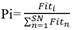(6)

where fiti is the fitness value of the solution xi (Power).

The fitness of each new produced candidate solution vij is com-pared with that of its old one. If the new solution has an equal or better fitness than the old solution, it replaces the old one in the memory. Otherwise, the old one is retained in the memory. In other words, a greedy selection mechanism is employed in the selection operation between the old and the candidate one.

4. At the end of each search cycle, if the fitness of a solution cannot be improved and the predetermined number of trials, which is called "limit", is exhausted, then the solution will be abandoned by scout bee and a new solution is randomly searched.

The main advantage of the ABC-based algorithm is that it does not require expending more effort in tuning the control parameters, as in the case of GA [56-59], and other evolutionary algorithm. This feature marks the proposed ABC-based algorithm as being advantageous for implementation.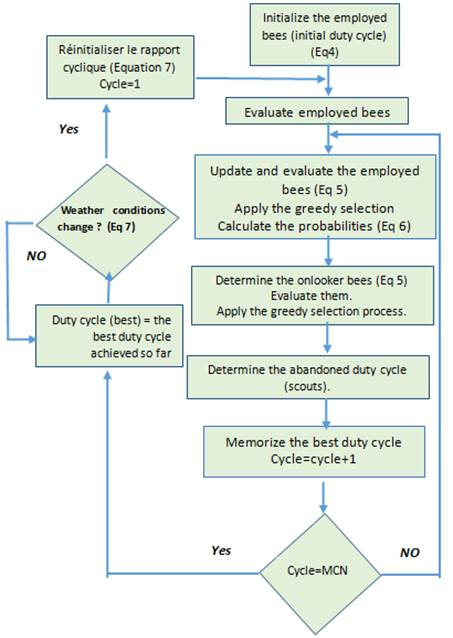Figure 6. Flowchart of the proposed ABC-based MPPT.

3. Results and Discussions

In this section we will compare the performance of P&O which has a fixed Δd (representing the step size) to those of the ABC.

For T=25°C and G=1000W /m²

1)  P&O algorithm with a fixed step size Δd=0.025 and Ts=0.002s: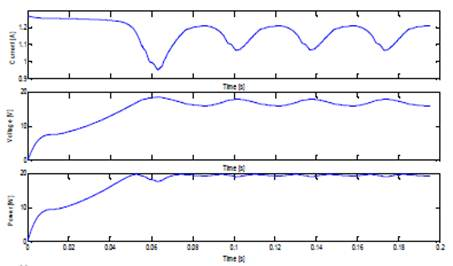Figure 7. Trend of IPV, VPV and PPV for P&O algorithm.

As we observe that the P&O with a fixed Δd=0.02, oscillate at the steady state around the MPP. These oscillations is due to the unstable values of duty cycle « d » which does not allow the algorithm to lead the PV module to reach the maximum power point effectively.

2)  ABC Algorithm: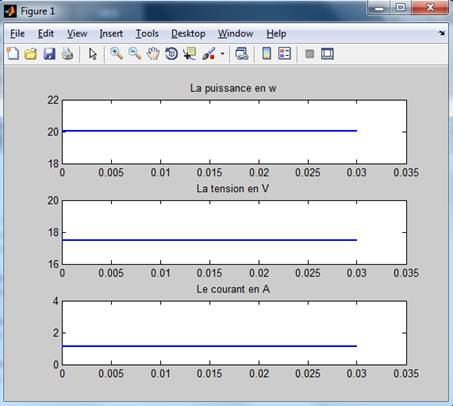Figure 8. Trend of IPV, IPV and PPV for ABC method.

The ABC algorithm find the maximum power point (MPP) at t=0s while P&O find the value of the same point at t= 0.053s and the ABC can track the MPP quickly and effectively without any oscillations in the steady state.

During a change in temperature or irradiation, the ABC algorithm resets (we control the fluctuation of weather conditions through equation 7) then converges to the new maximum power which makes our more robust algorithm.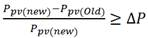(7)

4. Conclusion

In this paper we clearly see that the ABC algorithm which is considered as an intelligent control outperforms the method of MPPT P&O in tracking the MPP. We have shown through the simulation of photovoltaic system in simulink that the technique of ABC under variable irradiation conditions is more robust and has satisfactory results. In addition, the proposed algorithm requires only two control parameters; its convergence is not dependent on the initial condition.

Beside all of that, it provides just one optimal value of duty cycle, in contrast P&O find many values of duty cycle which causes in the end of their convergence an instability and oscillations in the steady state.

Acknowledgements

This work returns the framework of the research project SISA1 "Mini intelligent Power plant" began between research center SISA and our University. We are anxious to think the Hassan II University of Casablanca for the financing of this project.

References

1. E. M. Ahmed and M. Shoyama, "Variable Step Size Maximum Power Point Tracker Using a Single Variable for Stand-alone Battery Storage PV Systems," Journal of Power Electronics, Vol. 11, No. 2, March 2011, pp. 218-227.
2. N. Femia, G. Petrone, G. Spagnuolo and M. Vitelli, "Optimization of Perturb and Observe Maximum Power Point Tracking Method," IEEE TRANSACTIONS ON POWER ELECTRONICS, Vol. 20, No. 4, July 2005, pp. 963-973.
3. S. Mekhilef, R. Saidur, and A. Safari, "A review on solar energy use in industries," Renew. Sustain. Energy Rev., vol. 15, no. 4, pp. 1777–1790, May 2011.
4. S. Mekhilef, A. Safari, W. E. S. Mustaffa, R. Saidur, R. Omar, and M. A. A. Younis, "Solar energy in Malaysia: Current state and prospects, "Renew. Sustain. Energy Rev., vol. 16, no. 1, pp.386–396, Jan. 2012.
5. M. A.Eltawil and Z. Zhao, "MPPT techniques for photovoltaic applications," Renewable and Sustainable Energy Reviews, Vol. 25, 2013, pp. 793-813.
6. Salhi, Mohamed, and Rachid El-Bachtri. "Maximum Power Point Tracker using Fuzzy Control for Photovoltaic System." International Journal of Research and Reviews in Electrical and Computer Engineering 1.2 (2011): 69-75.
7. Guang Yi Cao, «Mathematical Models of Dc-Dc Converters», Journal of Zhejiang University, pp263-270, China, 2009
8. V. Tereshko, T. Lee, How information mapping patterns determine foraging behaviour of a honeybee colony, Open Syst. Inf. Dyn. 9 (2002) 181–193.
9. V. Tereshko, A. Loengarov, Collective decision-making in honeybee foraging dynamics, Comput. Inf. Syst. J. 9 (2005) 1–7.
10. A. S. Oshaba, E. S. Ali and S. M. Abd Elazim "Artificial Bee Colony Algorithm Based Maximum Power Point Tracking in Photovoltaic System"WSEAS TRANSACTIONS on POWER SYSTEMS(2015) 126-127.

 Contents 1. 2. 2.1. 2.2. 2.3. 3. 4.
Article ToolsAbstractPDF(1245K)
Follow on us
PUBLICATION SERVICE
JOIN US
RESOURCES
SPECIAL SERVICES
ADDRESS
Science Publishing Group
548 FASHION AVENUE
NEW YORK, NY 10018
U.S.A.
Tel: (001)347-688-8931